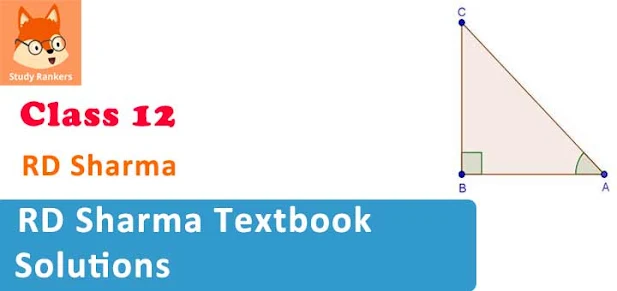# RD Sharma Textbook Solutions for Class 12 Maths

RD Sharma Solutions for Class 12 Maths is very important in building key concepts in the students. These solutions have been made by experts based on the textbook of RD Sharma. Solutions provided on this website are explained step-by-step to make it easier for students to understand. We have also solved illustrative examples and MCQ provided in the textbook. A complete solutions to RD Sharma class 12 Maths is given on this website.Exercises in each chapter have been updated with new illustrations and problems. A clear and concise explanation of all concepts and definitions has been given in each chapter along with suitable illustrative examples.

Features of RD Sharma Solutions for Class 12 Maths:
• Solutions are provided stepwise.
• Complete solutions are given with indicative formulas.
• Easy way to solve problems along with concepts.
• Detailed explanations to each and every questions.
Class 12 RD Sharma Solutions is free resource which can be studied by students to achieve growth in their life. The lessons in RD Sharma is designed in such a way that it boost the knowledge and confidence.

## Chapterwise RD Solutions for Class 12 Maths

• Chapter 1: Relations
• Chapter 2: Functions
• Chapter 3: Binary Operations
• Chapter 4: Inverse Trigonometric Functions
• Chapter 5: Algebra of Matrices
• Chapter 6: Determinants
• Chapter 7: Adjoint and Inverse of a Matrix
• Chapter 8: Solution of Simultaneous Linear Equations
• Chapter 9: Continuity
• Chapter 10: Differentiability
• Chapter 11: Differentiation
• Chapter 12: Higher Order Derivatives
• Chapter 13: Derivative as a Rate Measurer
• Chapter 14: Differentials, Errors and Approximations
• Chapter 15: Mean Value Theorems
• Chapter 16: Tangents and Normals
• Chapter 17: Increasing and Decreasing Functions
• Chapter 18: Maxima and Minima
• Chapter 19: Indefinite Integrals
• Chapter 20: Definite Integrals
• Chapter 21: Areas of Bounded Regions
• Chapter 22: Differential Equations
• Chapter 23 Algebra of Vectors
• Chapter 24: Scalar Or Dot Product
• Chapter 25: Vector or Cross Product
• Chapter 26: Scalar Triple Product
• Chapter 27: Direction Cosines and Direction Ratios
• Chapter 28 Straight line in space
• Chapter 29: The plane
• Chapter 30: Linear programming
• Chapter 31: Probability
• Chapter 32: Mean and variance of a random variable
• Chapter 33: Binomial Distribution

Solutions for Class 12 RD Sharma is based on the latest syllabus and patterns provided by CBSE. Students can also check out Class 12 Maths NCERT Solutions to understand more about the course and topics which they need to study. Textbook of RD Sharma is also very beneficial in competitive exams.

Students who are preparing for JEE and other engineering exams must solve Class 12 RD Sharma Solutions to excel in the examinations. These stepwise solutions are solved in such a way that it became very easy for a student to understand the question properly. Also, this textbook has two level of questions based on the difficulty to solve it. At the end of the chapter, MCQ's are also given.